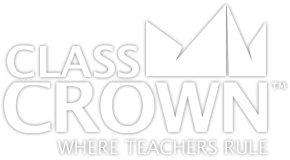## 2nd Grade Math Worksheets: Pack 1

Skills included in this pack:
• Solving addition word problems within 100
• Solving two-step addition word problems
• Solving two-step word problems involving addition and subtraction
• Subtracting with 100
• Solving subtraction word problems within 100
• Adding and subtracting within 20
• Adding and subtracting within 20 (using a symbol to stand in as a number)
• Finding even and odd numbers of shapes
• Creating even equations
• Creating addition equations from arrays
• Creating multiplication equations from arrays
• Measuring in inches
• Comparing two measurements
• Estimating measurements
• Measuring to determine the difference in length
• Solving word problems involving lengths in the same unit
• Adding and subtracting with number lines
• Telling time from analog clocks to the half hour
• Telling time from analog clocks to the quarter hour
• Telling time from analog clocks in 5 minute increments
• Counting money, pennies and dimes
• Counting money, nickels and dimes
• Counting money, dimes, nickels, and pennies
• Counting money, quarters, dimes, nickels, and pennies
• Counting money, dollars and cents
• Counting money word problems
• Measuring and creating line plots
+ See More
Free Sample Pages Available## 2nd Grade Math Worksheets: Pack 2

Skills included in this pack:
• Creating groups of 100
• Identifying quantity with 100 blocks
• Understanding 3-digit places
• Identifying groups of 100
• Counting up
• Counting up by fives, tens, and hundreds
• Counting up by tens and hundreds
• Converting expanded form to numeric within 1,000
• Converting numeric form to expanded form within 1,000
• Converting numeric form to word form within 1,000
• Converting word form to numeric form within 1,000
• Comparing 2-digit numbers
• Comparing 3-digit numbers
• Adding within 100, no regrouping
• Adding within 100, with regrouping
• Subtracting within 100, no regrouping
• Subtracting within 100, with regrouping
• Subtracting across zero
• Adding and subtracting within 100, including variables
• Subtracting within 1,000
• Adding and subtracting within 1,000
• Adding and subtracting within 1,000, including variables
• Adding and subtracting 10 or 100 more
• Identifying shapes
• Partitioning rectangles
• Naming fractions
+ See More
Free Sample Pages Available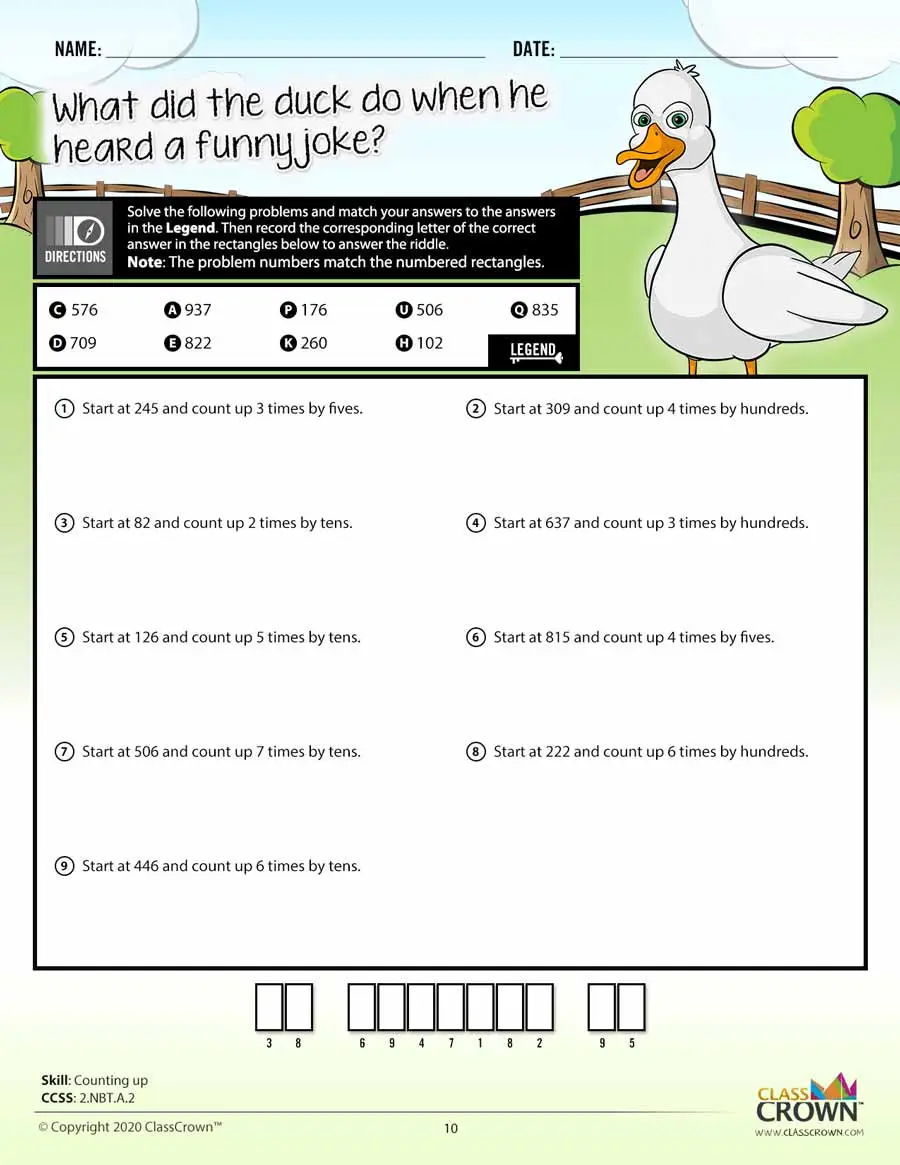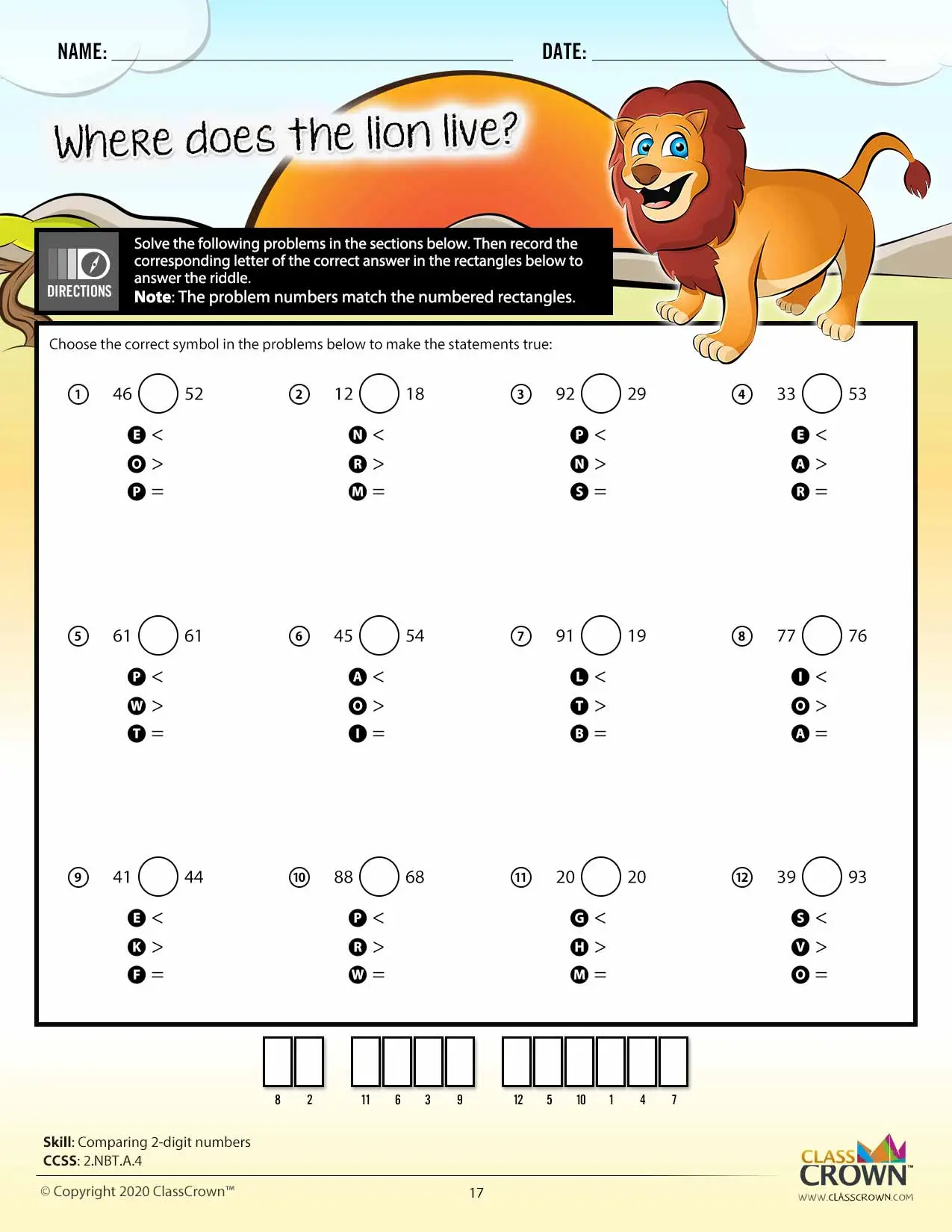Self Checking

Our math worksheets introduce a puzzle aspect to math, giving students immediate feedback as to whether or not they are solving problems correctly. If the answer to the riddle isn't spelled correctly, the student knows which problems he's made an error on.Fun Puzzle AspectImmediate Feedback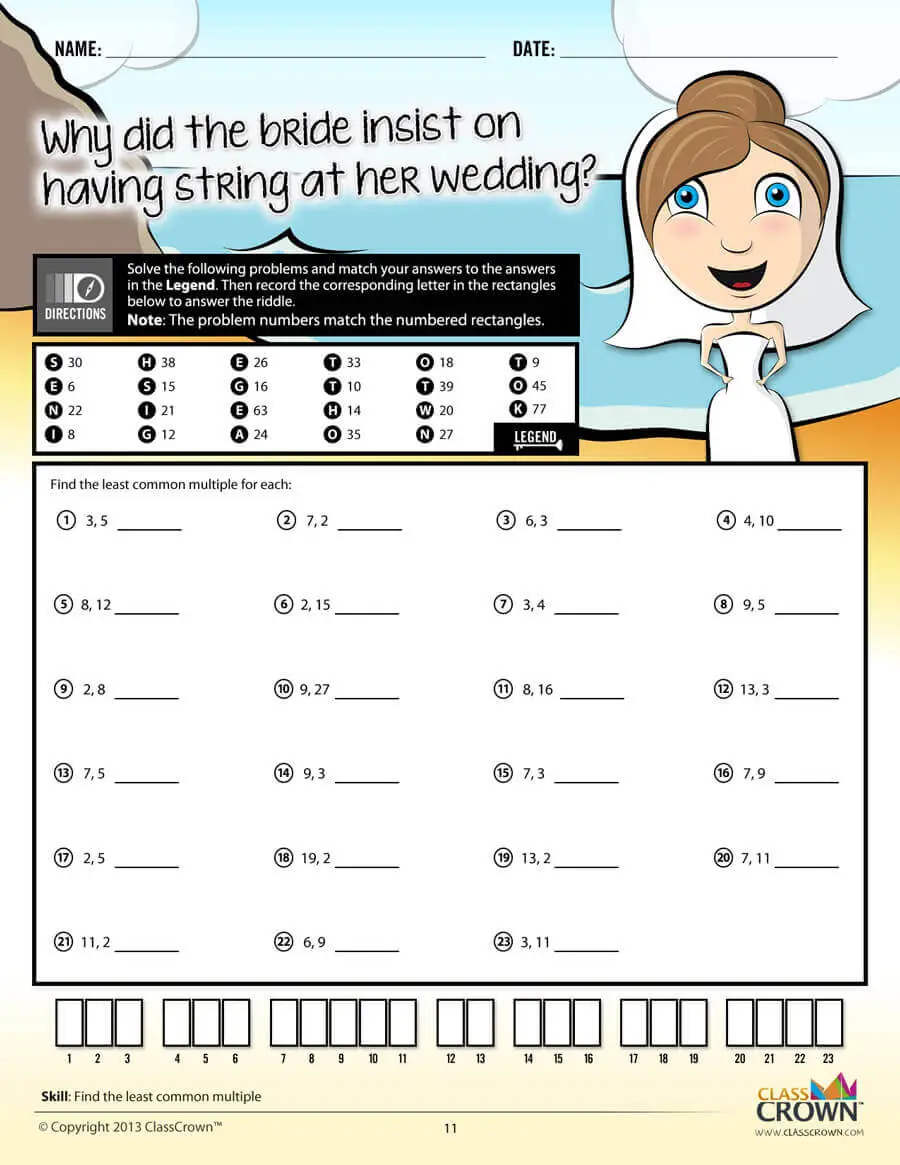Problem Solving Motivation

Each math worksheet contains a riddle that the student solves by completeing all the problems on the worksheet. This keeps kids motivated to complete each problem so that they can find the answer to the riddle.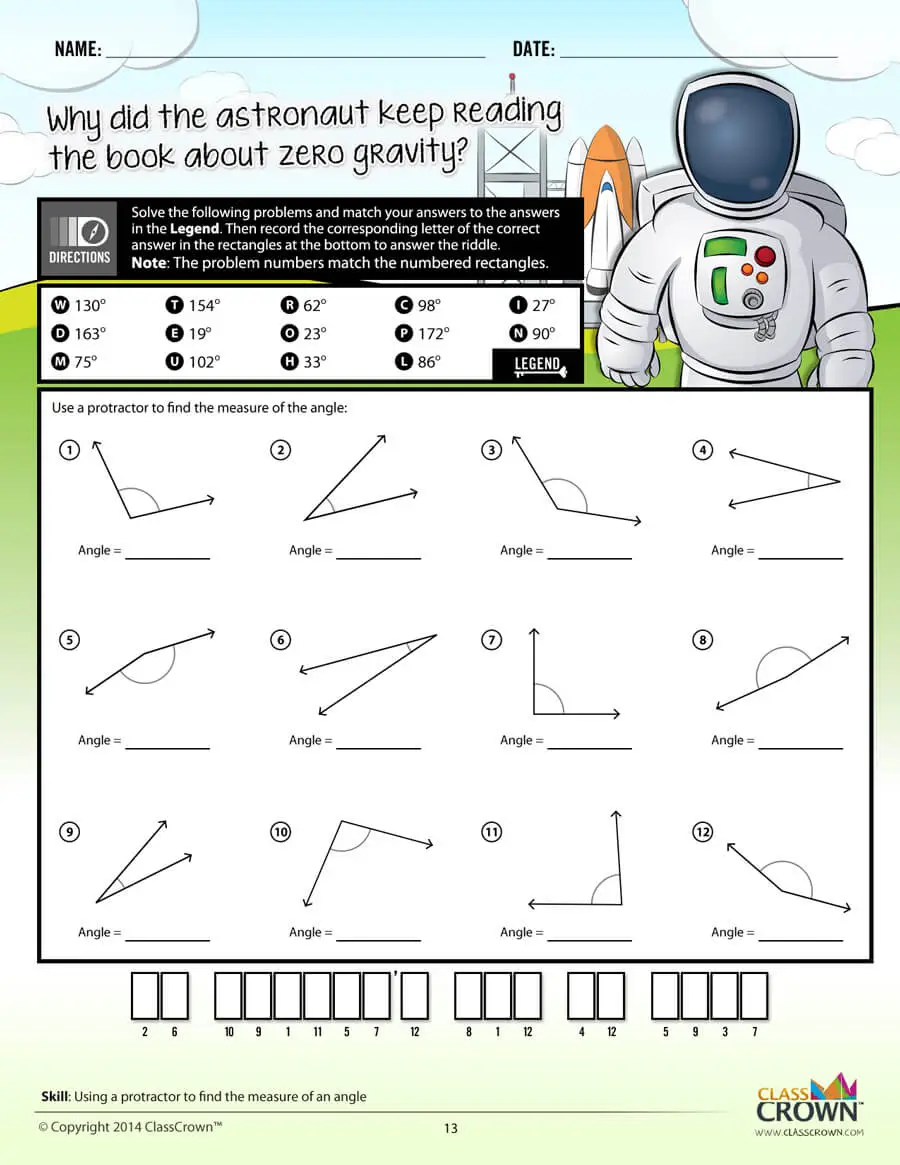Common Core Aligned

All our math worksheet packs are designed with Common Core in mind. That way you don’t have to worry about whether your math ciriculum is aligned or not when you incorpoate ClassCrown Riddle-Me-Worksheets in your lesson plans.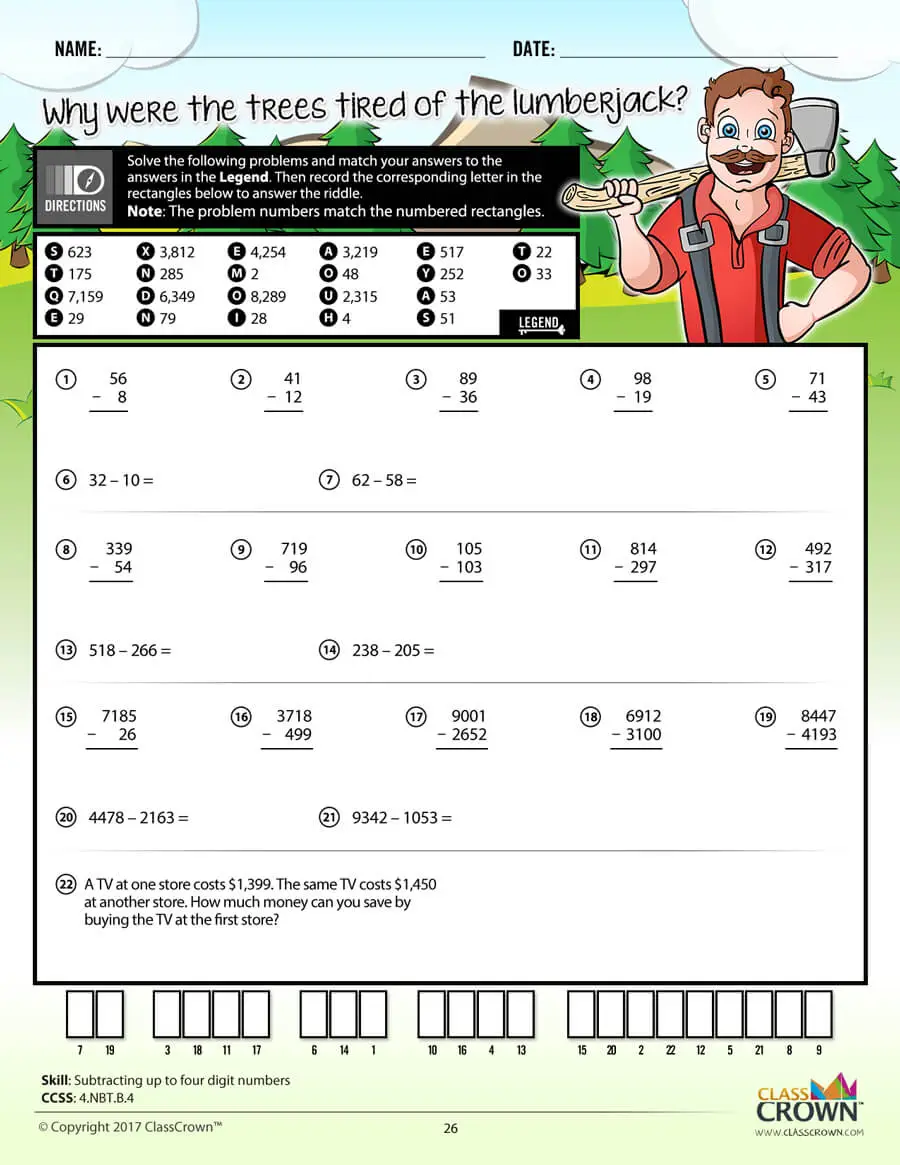High Quality Design

Each page of our math worksheets has been produced in high resolution at 144 dpi and designed in full, vibrant color for maximum quality. They look stunning whether you are printing in color or black and white.High Resolution
(144 dpi)Stunning Color & Clarity